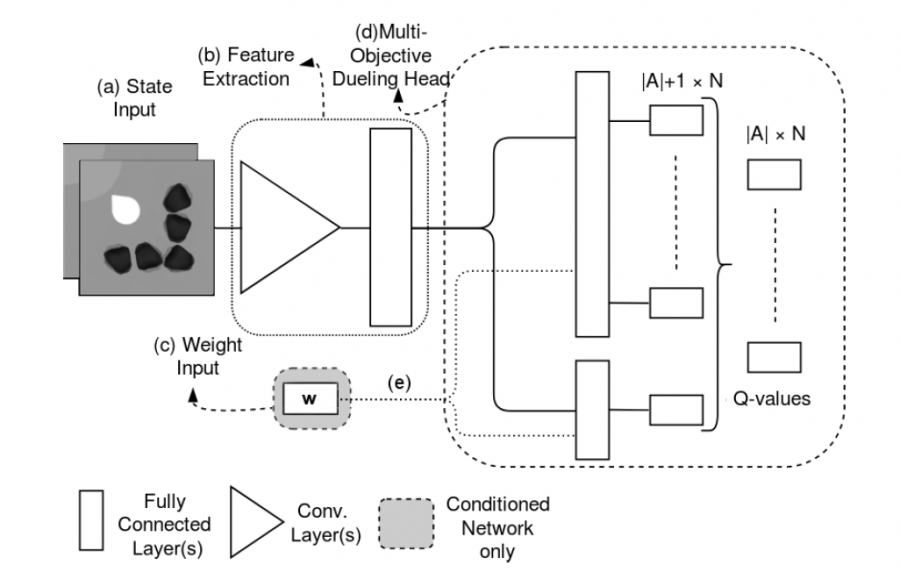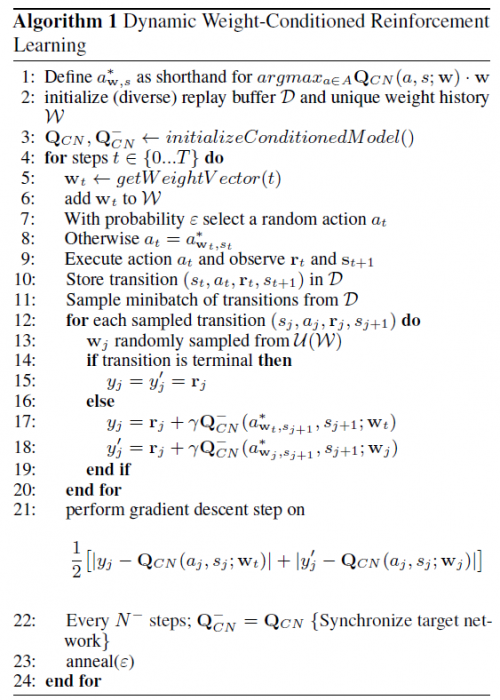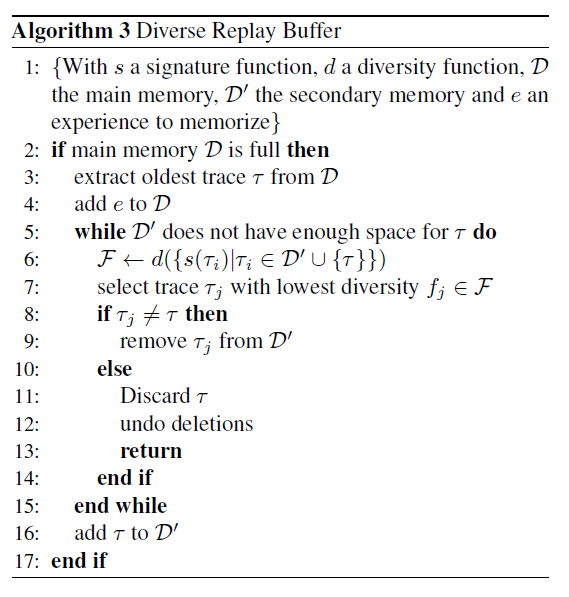# 单智能体强化学习算法

## Dynamic Weights in Multi-Objective Deep Reinforcement Learning

Posted by MY on August 10, 2020

## 二、解法$\begin{array}{l} \frac{1}{2}\left[\left|\mathbf{y}_{\mathbf{w}_{t}}^{(j)}-\mathbf{Q}_{C N}\left(a_{j}, s_{j} ; \mathbf{w}_{t}\right)\right|+\left|\mathbf{y}_{\mathbf{w}_{j}}^{(j)}-\mathbf{Q}_{C N}\left(a_{j}, s_{j} ; \mathbf{w}_{j}\right)\right|\right] \\ \mathbf{y}_{\mathbf{w}}^{(j)}=\mathbf{r}_{j}+\gamma \mathbf{Q}_{C N}^{-}\left(\underset{a \in A}{\operatorname{argmax}} \mathbf{Q}_{C N}\left(a, s_{j+1} ; \mathbf{w}\right) \cdot \mathbf{w}, s_{j+1} ; \mathbf{w}\right) \end{array}$## 三、实验内容## 五、优点

##### Share